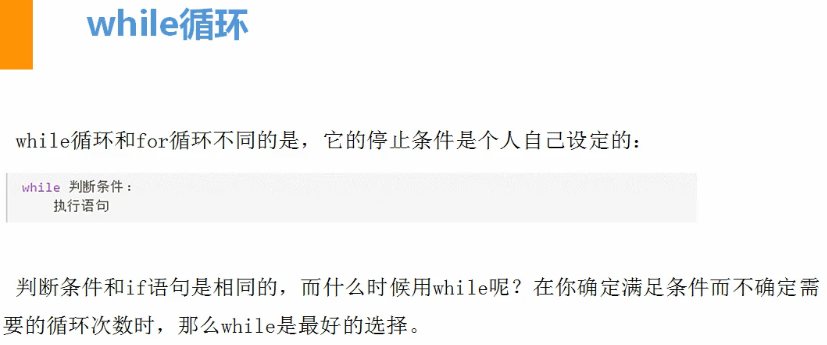出租广告位,需要合作请联系站长

+关注

## 1、while循环```使用while打印1.2.3.4.5.6.8.9.10      #快速注释Ctrl+？count = 1
while count <= 10:
if count == 7:
count = count + 1
pass  #表示过，不执行下面程序
else:
print(count)
count = count + 1
print('end')```

```count = 1
while count <= 10:
if count != 7:  #!=表示不等于
print(count)
count = count + 1
print('end')```

```错误示例
count = 1
while count <= 10 and count != 7:
print(count)
count = count + 1
print('end')```

### 关键字：break终止当前循环

continue #结束本次循环，直接开始下个循环

```while True:#True首字需大写，否则报错name 'true' is not defined（名称“true”没有定义）
print(666)
break #终止当前循环
print('end')```
```通过break实现1~10
while True:
print(count)
if count==10:
break
count = count+1
print('end')```

```while True:
print('你好')
while True:
print(666)
break```

### 关键字：continue本次循环如果遇到continue，则不再继续下面程序，而是回到while条件位置

```实现1234568910
count = 1
while count <= 10:
if count == 7:
count = count + 1
continue
else:
print(count)
count = count + 1
print('end')```

```count = 1
while count <= 10:
print(count)
count = count + 1
continue
else:#当不再满足while后的条件时，触发。或 条件=False
print('else代码块')
print('end')```
```count = 1
while True:
print(count)
if count == 10:
break
count = count + 1
else:#当不再满足while后的条件时，触发。或 条件=False
print('else代码块')
print('end')```

## 2、字符串格式化

#### %d:代指数字

```#字符串格式化存在的意义
name = input('姓名')
do = input('在干什么:')

template = "我是%s ,%s。"%(name,do,)
print(template)```

```template = "我是%s ,年龄%d，职业%s。"%('alex',25,'engineer',)
print(template)```

#### 特殊：当需要打印%时，要多加一个百分号（%%）

```name = 'alex'
template = '%s手机的电量是100%%'%(name)
print(template)```

```name = input('请输入姓名:')
age = input('请输入年龄:')
job = input('请输入职业:')
hobby = input('请输入爱好:')

msg = '''
---------info of alex li--------
name: %s
age: %s
job: %s
hobby: %s
---------end-------'''
print(msg%(name,age,job,hobby))```

## 3、运算符

```输出1-100内的所有偶数n=1
while n<101:
temp= n % 2#取模 - 返回除法的余数
if temp==0:
print(n)
else:
pass
n=n+1```
```求1-2+3-4+5...99的所有数的和
n=0
a=0 #a是之前所有数的总和
while n<100:
print(n)
temp=n%2
if temp==0:
a=a-n
else:
a=a+n
n=n+1

print(a)```

## Python算术运算符

+ 加 - 两个对象相加 a + b 输出结果 31
- 减 - 得到负数或是一个数减去另一个数 a - b 输出结果 -11
* 乘 - 两个数相乘或是返回一个被重复若干次的字符串 a * b 输出结果 210
/ 除 - x 除以 y b / a 输出结果 2.1
% 取模 - 返回除法的余数 b % a 输出结果 1
** 幂 - 返回x的y次幂 a**b 为10的21次方
// 取整除 - 向下取接近除数的整数
```>>> 9//2
4
>>> -9//2
-5```

## Python赋值运算符

= 简单的赋值运算符 c = a + b 将 a + b 的运算结果赋值为 c
+= 加法赋值运算符 c += a 等效于 c = c + a
-= 减法赋值运算符 c -= a 等效于 c = c - a
*= 乘法赋值运算符 c *= a 等效于 c = c * a
/= 除法赋值运算符 c /= a 等效于 c = c / a
%= 取模赋值运算符 c %= a 等效于 c = c % a
**= 幂赋值运算符 c **= a 等效于 c = c ** a
//= 取整除赋值运算符 c //= a 等效于 c = c // a
:= 海象运算符，可在表达式内部为变量赋值。Python3.8 版本新增运算符

```if (n := len(a)) > 10:
print(f"List is too long ({n} elements, expected <= 10)")```

## Python逻辑运算符

Python语言支持逻辑运算符，以下假设变量 a 为 10, b为 20:

and x and y 布尔"与" - 如果 x 为 False，x and y 返回 False，否则它返回 y 的计算值。  (a and b) 返回 20。
or x or y 布尔"或" - 如果 x 是 True，它返回 x 的值，否则它返回 y 的计算值。 (a or b) 返回 10。
not not x 布尔"非" - 如果 x 为 True，返回 False 。如果 x 为 False，它返回 True。 not(a and b) 返回 False
```# 对于or，如果遇到
value1 = 0 or 1
value2 = 8 or 10
value3 = 0 or 9 or 8
print(value)
# 第一个值如果是转换成布尔值如果是真，则value=第一值；
# 第一个值如果是转换成布尔值如果是假，则value=第二值；
# 如果有多个or条件，则从左到右依次进行上述流程。

# 对于and,如果遇到
# 第一个值如果是转换成布尔值如果是True，则value=第二值；
# 第一个值如果是转换成布尔值如果是False，则value=第一值；
# 如果有多个and条件，则从左到右依次进行上述流程。
value1 = 1 and 9
value2 = 1 and 0
value3 = 0 and 7
value4 = 0 and ""
value5 = 1 and 0 and 9
print(value)

#综合
#先看and在看or;如果有括号先算括号内容。优先级关系()>not>and>or,同一优先级从左往右计算。
value1 = 1 and 9 or 0 and 8
print(value)```

ascii:

unicode:

ecs2

ecs4

utf-8:

utf-16:

23 0

pdf(new) 更多>